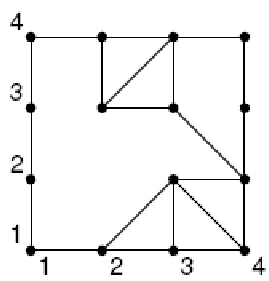Online JudgeProblem SetAuthorsOnline ContestsUser
Web Board
F.A.Qs
Statistical Charts
Problems
Submit Problem
Online Status
Prob.ID:
Register
Authors ranklist
Current Contest
Past Contests
Scheduled Contests
Award Contest
Register
Language:
Connect the Dots
 Time Limit: 1000MS Memory Limit: 65536K Total Submissions: 202 Accepted: 24

Description

Given a rectangular region of the plane which has been divided into polygons, decide what the maximum number of edges that any of the polygons has. For example, if the region is divided into triangles, then the maximal number is 3. If the region is divided into, say, 7 triangles and 2 squares, then the maximal number is 4.

There will be a list of pairs of (x, y) coordinates: Each (x, y) coordinates represents a vertex, and consequently each pair of coordinates represents an edge. The edges collectively divide the rectangular region naturally into disjoint ``n-gons". (Explanation: A triangle is a 3-gon, a square is an example of a 4-gon, etc.) That is, the boundary of each n-gon is a union of edges.

Figure 1 is a sketch of an example 3 x 3 rectangular region divided into five 3-gons, a 5-gon and a 10-gon.The pairs of coordinates representing the edges of the 5-gon would be:

{((3, 4),(4, 4)),((4, 4),(4, 3)),((3, 4),(3, 3)),((3, 3),(4, 2)),((4, 3),(4, 2))}

The maximal number of edges in this example is 10. That is, this example has a 10-gon.

Input

The input consists of multiple test cases. The first line of each test case will consist of an integer n representing the number of edges. Each test case will then contain n pairs of (x, y) (|x|, |y| ≤ 100) coordinates where x, yZ which represent edges dividing a rectangular region into n-gons. Each integer will be separated by a white space. Overlapping edges will not be specified in the input. The input will terminate for n = 0.

Output

The output should be an integer describing the maximal number of edges that any of those n-gon has. Separate each test case with a new line.

Sample Input

```21
1 1 1 2
1 2 1 3
1 3 1 4
1 1 2 1
2 1 3 1
3 1 4 1
1 4 2 4
2 4 3 4
3 4 4 4
4 1 4 2
4 2 4 3
4 3 4 4
2 3 2 4
2 3 3 4
2 3 3 3
3 3 3 4
3 3 4 2
2 1 3 2
3 2 3 1
4 1 3 2
3 2 4 2
0```

Sample Output

`10`

Source

[Submit]   [Go Back]   [Status]   [Discuss]Home PageGo BackTo top